# ISEE Middle Level Quantitative : How to multiply fractions

## Example Questions

← Previous 1

### Example Question #1 : How To Multiply Fractions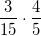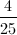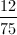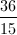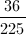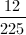Explanation:

When multiplying fractions you simply multiply the numerators to find the numerator of the product, and multiply the denominators to find the denominator of the product.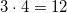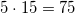So, our product is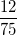.

Since both of these numbers are divisible by three, we simplify.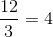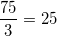Our final answer is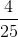.

### Example Question #2 : How To Multiply Fractions

Which is the greater quantity?

(a)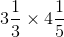(b)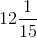It is impossible to tell from the information given

(b) is greater

(a) and (b) are equal

(a) is greater

(a) is greater

Explanation:

Rewrite the mixed numbers as improper fractions, then multiply across: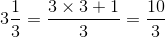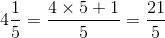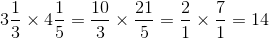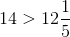### Example Question #3 : How To Multiply Fractions

Which is the greater quantity?

(a)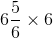(b)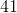(a) is greater

(a) and (b) are equal

(b) is greater

It is impossible to tell from the information given

(a) and (b) are equal

Explanation:

Rewrite the factors as improper fractions, then multiply across: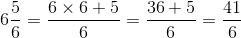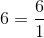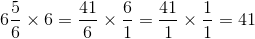### Example Question #4 : How To Multiply Fractions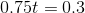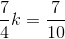Which is the greater quantity?

(a)(b)(a) and (b) are equal

(a) is greater

(b) is greater

It is impossible to tell from the information given

(a) and (b) are equal

Explanation:

(a)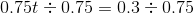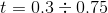Divide by moving the decimal point right two places in both numbers: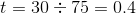(b)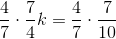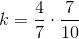Cross-cancel: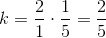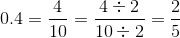, so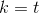### Example Question #5 : How To Multiply Fractions

Which is the greater quantity?

(A)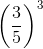(B)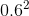It is impossible to determine which is greater from the information given

(A) is greater

(B) is greater

(A) and (B) are equal

(B) is greater

Explanation: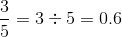, so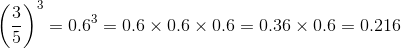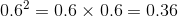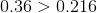, so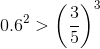, making (B) greater.

### Example Question #6 : How To Multiply Fractions

Which is the greater quantity?

(A)(B)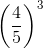(A) and (B) are equal

It is impossible to determine which is greater from the information given

(B) is greater

(A) is greater

(B) is greater

Explanation: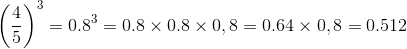Since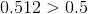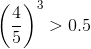, so (B) is greater.

### Example Question #7 : How To Multiply Fractions

Which is the greater quantity?

(A)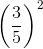(B)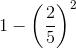(B) is greater

(A) and (B) are equal

It is impossible to determine which is greater from the information given

(A) is greater

(B) is greater

Explanation: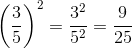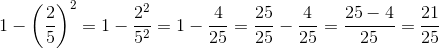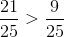, so (B) is greater

### Example Question #8 : How To Multiply Fractions

Which is the greater quantity?

(A)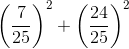(B)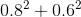(A) is greater

It is impossible to determine which is greater from the information given

(A) and (B) are equal

(B) is greater

(A) and (B) are equal

Explanation: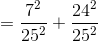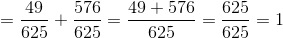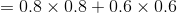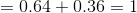The expressions are equal.

### Example Question #9 : How To Multiply Fractions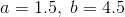Which is the greater quantity?

(A)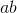(B)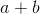It is impossible to determine which is greater from the information given

(A) is greater

(A) and (B) are equal

(B) is greater

(A) is greater

Explanation:

Solve for (A):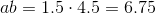Solve for (B):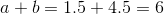Since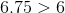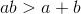and (A) is greater.

### Example Question #10 : How To Multiply Fractions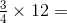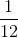Explanation:

When dealing with problems that involve fractions and whole numbers, first convert your whole number into a fraction. You can easily do this by putting the whole number over 1.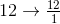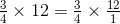When multiplying, multiply the numerators by each other and the denominators by each other.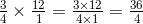Always reduce a fraction when possible. In this case, the numerator and denominator of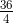are both divisible by 4. So, it reduces to 9.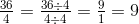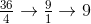← Previous 1